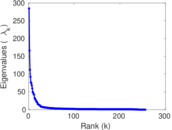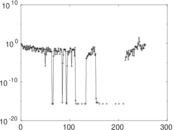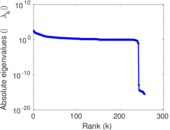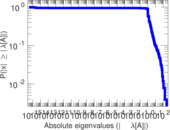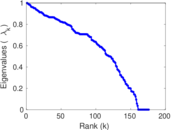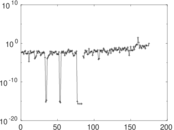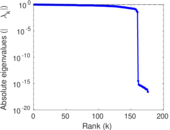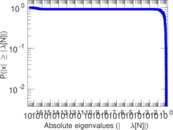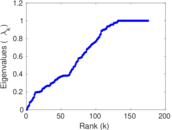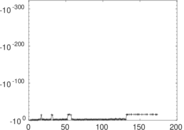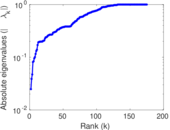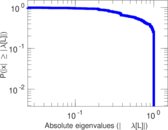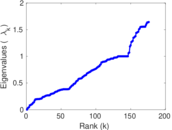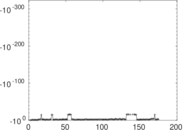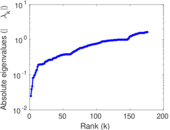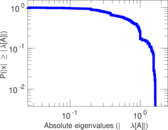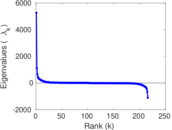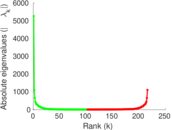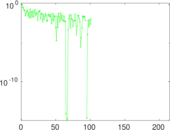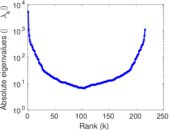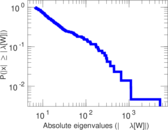# Wikibooks edits (ka)

This is the bipartite edit network of the Georgian Wikibooks. It contains users and pages from the Georgian Wikibooks, connected by edit events. Each edge represents an edit. The dataset includes the timestamp of each edit.

 Code `bka` Internal name `edit-kawikibooks` Name Wikibooks edits (ka) Data source http://dumps.wikimedia.org/ AvailabilityDataset is available for download Consistency checkDataset passed all tests Category Authorship network Dataset timestamp 2017-10-20 Node meaning User, article Edge meaning Edit Network formatBipartite, undirected Edge typeUnweighted, multiple edges Temporal dataEdges are annotated with timestamps

## Statistics

 Size n = 5,267 Left size n1 = 258 Right size n2 = 5,009 Volume m = 12,212 Unique edge count m̿ = 6,465 Wedge count s = 4,214,384 Claw count z = 3,014,264,684 Cross count x = 1,773,540,974,581 Square count q = 166,179 4-Tour count T4 = 18,200,034 Maximum degree dmax = 4,272 Maximum left degree d1max = 4,272 Maximum right degree d2max = 168 Average degree d = 4.637 17 Average left degree d1 = 47.333 3 Average right degree d2 = 2.438 01 Fill p = 0.005 002 62 Average edge multiplicity m̃ = 1.888 94 Size of LCC N = 4,994 Diameter δ = 13 50-Percentile effective diameter δ0.5 = 3.349 57 90-Percentile effective diameter δ0.9 = 4.381 25 Median distance δM = 4 Mean distance δm = 3.658 98 Gini coefficient G = 0.754 878 Balanced inequality ratio P = 0.187 439 Left balanced inequality ratio P1 = 0.078 611 2 Right balanced inequality ratio P2 = 0.288 814 Relative edge distribution entropy Her = 0.718 189 Power law exponent γ = 6.107 56 Tail power law exponent γt = 2.841 00 Tail power law exponent with p γ3 = 2.841 00 p-value p = 0.000 00 Left tail power law exponent with p γ3,1 = 1.661 00 Left p-value p1 = 0.786 000 Right tail power law exponent with p γ3,2 = 3.031 00 Right p-value p2 = 0.000 00 Degree assortativity ρ = −0.209 748 Degree assortativity p-value pρ = 3.401 04 × 10−65 Spectral norm α = 284.651 Algebraic connectivity a = 0.024 784 6 Spectral separation |λ1[A] / λ2[A]| = 1.712 22 Controllability C = 4,777 Relative controllability Cr = 0.908 002

## Plots

### Fruchterman–Reingold graph drawing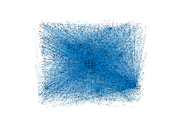### Degree distribution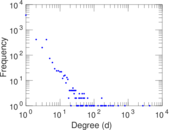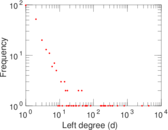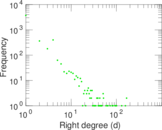### Cumulative degree distribution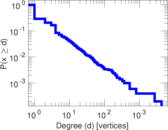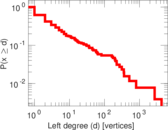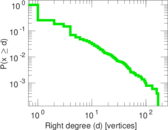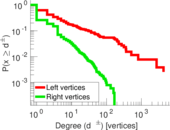### Lorenz curve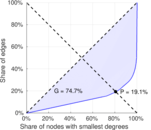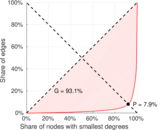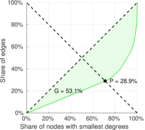### Spectral distribution of the adjacency matrix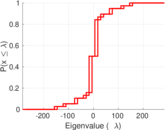### Spectral distribution of the normalized adjacency matrix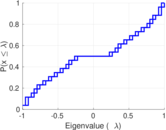### Spectral distribution of the Laplacian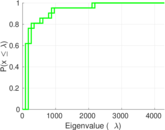### Spectral graph drawing based on the adjacency matrix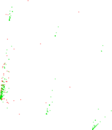### Spectral graph drawing based on the Laplacian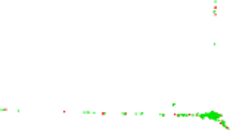### Spectral graph drawing based on the normalized adjacency matrix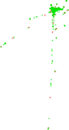### Degree assortativity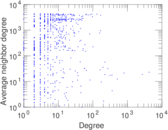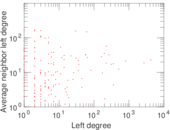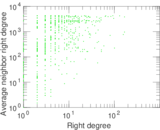### Zipf plot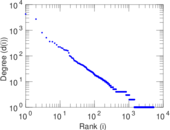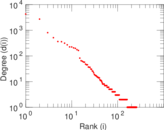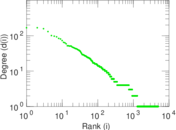### Hop distribution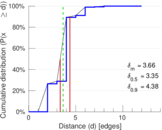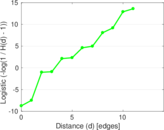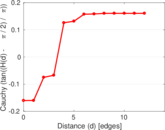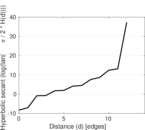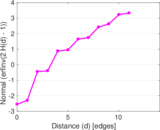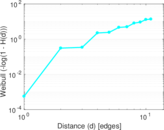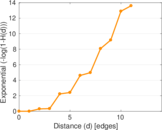### Double Laplacian graph drawing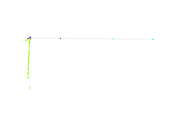### Delaunay graph drawing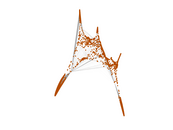### Edge weight/multiplicity distribution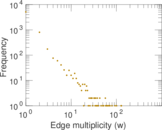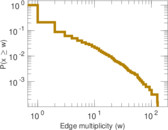### Temporal distribution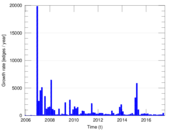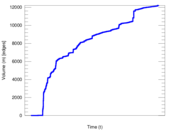### Temporal hop distribution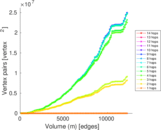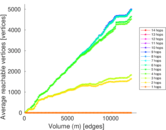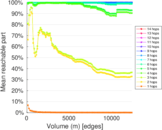### Diameter/density evolution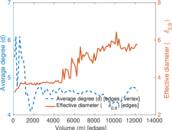### Matrix decompositions plots